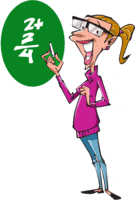# Math Worksheets Land

Math Worksheets For All Ages

# Math Worksheets Land

Math Worksheets For All Ages

Home > Math Topics > Addition >

These worksheets help students review all their addition operation skills in a wide array of topics. When we use the word “mixed” we are referring to the number length. This means that the problems on these sheets do not follow a standard pattern. You may find single digits being added to six or seven digit values and those are right next to a problem that involves finding the sum of a three and two digit value. This is great for reviewing and it is also great just to give to kids when you have extra time to fill. You can never review these skills enough. You will use them often in adulthood on a daily basis.

### Aligned Standard: 2.NBT.B.7

• Answer Keys - These are for all the unlocked materials above.

### Homework Sheets

These are fun and inviting to use as review packets.

### Practice Worksheets

Make sure to follow the progression that I have created it will help students grasp it faster.

### Math Skill Quizzes

We run the gamut up to three integers and three addends.

• Quiz 1 - The triple integer problems are a bit more difficult.
• Quiz 2 - These are much larger sums than previous problems.
• Quiz 3 - A solid way to end this topic off.

### How Do You Master Addition?Nothing is scarier for the kids and their parents than the art of learning mathematics. Do you remember the time when you were a little kid, staring at a blank paper trying to work through that basic mathematical addition sum? Luckily your students do not have to go through the same struggle trying to tackle the basic mathematical operations. If you provide them with a logical approach to learning these skills it is much easier.

Math Facts - It all begins with laying a solid foundation in single digit addition math facts. Students should have that down automatically and progress to not use their fingers. This can be done through math fact cards and is super helpful for advanced addition.

Skip Counting - Once those math facts are concrete, master the art of skip counting. Skip counting, which is normally in increments of 2s, 5s, 10s etc. This is fun to do and saves a lot of time. It helps kids to understand the patterns in counting.

Add 10s Places - Don't add 16 or 17 at once. Add the 10s place first and then add the remaining. This is a more advanced tip that you can use when you a solid with your math facts.

Add Doubles and Near Doubles - Adding doubles means twice the value of a number is added to the real number. Adding near double refers to adding the double then adding one more. Doubles are the natural progression before you move on to multiplication.

Rounding Helps Make It Quicker - A quick way to do this would be to round the value and add them. Then go back and see how you rounded them to process the calculation. This is a great habit to get into and can be applied to other operations, but I would make sure that you master it first. You may be adding 39 and 187 together. Using this method, we would round it as such: 40 (We added 1) + 190 (we added 3). So collectively (1 + 3 = 4), whatever our answer is, we will need to subtract 4 from the final answer. 40 + 190 = 230. We subtract 4 and get 226. That was a lot faster, wasn't it?

Practice, practice, and practice.

Unlock all the answers, worksheets, homework, tests and more!
Save Tons of Time! Make My Life Easier Now

## Thanks and Don't Forget To Tell Your Friends!

I would appreciate everyone letting me know if you find any errors. I'm getting a little older these days and my eyes are going. Please contact me, to let me know. I'll fix it ASAP.